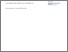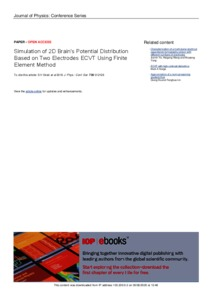# Simulation of 2D Brain's Potential Distribution Based on Two Electrodes ECVT Using Finite Element Method

Sirait, SH dan Edison, Rizki Edmi dan Baidillah, MR dan Taruno, WP dan Haryanto, F (2016) Simulation of 2D Brain's Potential Distribution Based on Two Electrodes ECVT Using Finite Element Method. Journal of Physics: Conference Series, 739. ISSN 1742-6596Preview Text Simulation of 2D Brains Potential Distribution Based on Two Electrodes ECVT using Finite Element Method.pdf Download (1MB) | Preview
Official URL: https://doi.org/10.1088/1742-6596/739/1/012126

## Abstract

The aim of this study is to simulate the potential distribution of 2D brain geometry based on two electrodes ECVT. ECVT (electrical capacitance tomography) is a tomography modality which produces dielectric distribution image of a subject from several capacitance electrodes measurements. This study begins by producing the geometry of 2D brain based on MRI image and then setting the boundary conditions on the boundaries of the geometry. The values of boundary conditions follow the potential values used in two electrodes brain ECVT, and for this reason the first boundary is set to 20 volt and 2.5 MHz signal and another boundary is set to ground. Poisson equation is implemented as the governing equation in the 2D brain geometry and finite element method is used to solve the equation. Simulated Hodgkin-Huxley action potential is applied as disturbance potential in the geometry. We divide this study into two which comprises simulation without disturbance potential and simulation with disturbance potential. From this study, each of time dependent potential distributions from non-disturbance and disturbance potential of the 2D brain geometry has been generated.

Item Type: Article Q Science > Q Science (General) Rizki Edmi Edison 15 Jun 2020 05:42 15 Jun 2020 05:42 http://repository.uhamka.ac.id/id/eprint/993View Item